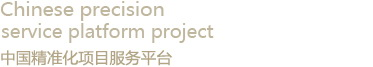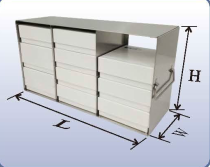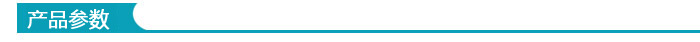# 存放2英寸、3英寸和3.75英寸高标准盒的立式冰箱

 产品名称：存放2英寸、3英寸和3.75英寸高标准盒的立式冰箱 产品型号：UF-306 UF-308 UF-312L UF-406 UF-408 UF-412L UF-506 UF-508 UF-512L 生产厂家：美国精骐•  型号 尺寸 (L×H×W) 容量 2" 3" 2"and 3" UF-306 415×159×140 mm 3×3=9 3×2=6 3×1+3×1 UF-308 415×210×140 mm 3×4=12 3×2=6 3×1+3×2 UF-312L 415×315×143 mm 3×6=18 3×4=12 3×3+3×2 UF-406 550×159×140 mm 4×3=12 4×2=8 4×1+4×1 UF-408 550×210×140 mm 4×4=16 4×2=8 4×1+4×2 UF-412L 550×315×143 mm 4×6=24 4×3=12 4×3+4×2 UF-506 679×159×140 mm 5×3=15 5×2=10 5×1+5×1 UF-508 679×210×140 mm 5×4=20 5×2=10 5×1+5×2 UF-512L 679×315×143 mm 5×6=30 5×3=15 5×3+5×2